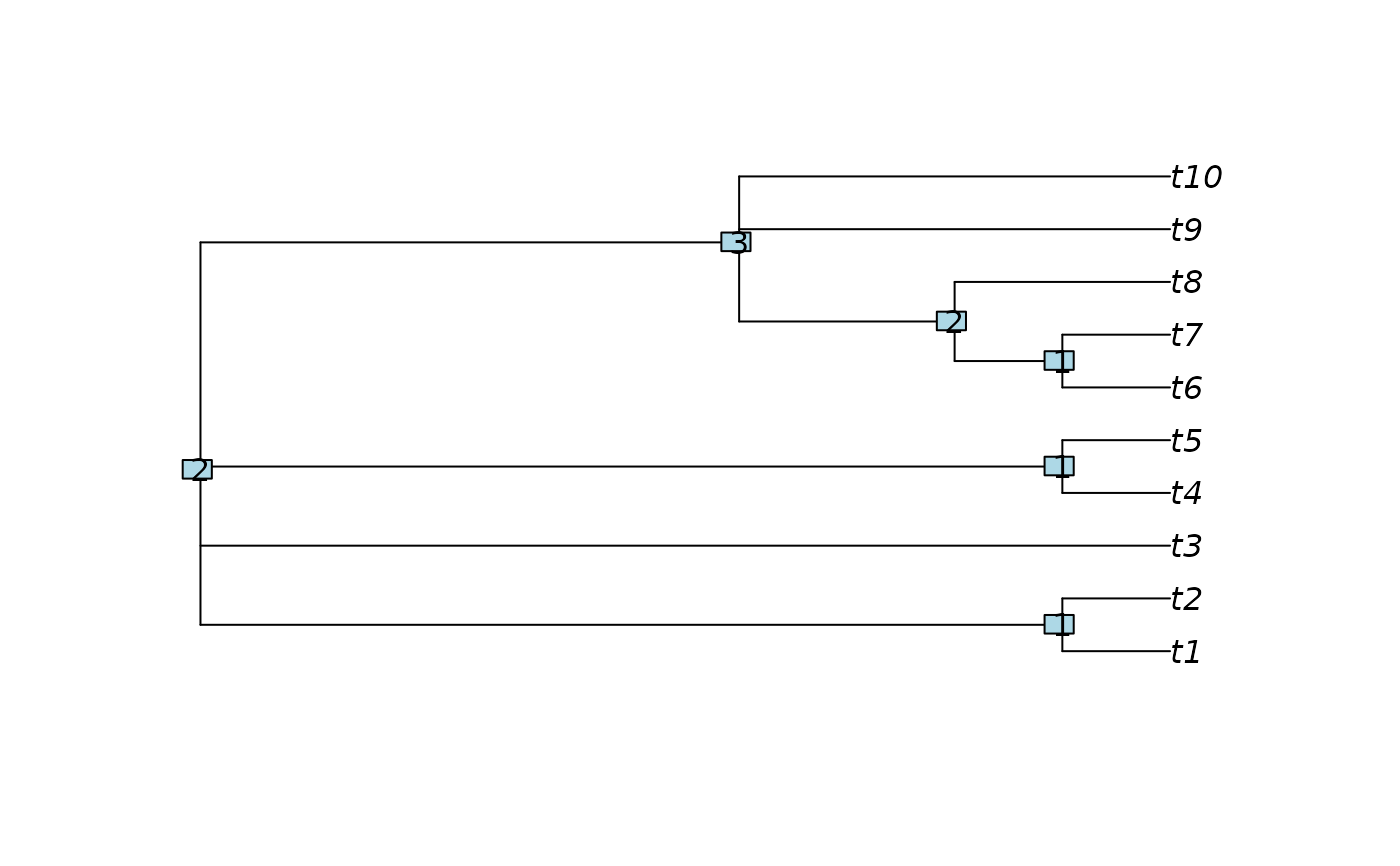NodeDepth() evaluates how 'deep' each node is within a tree.

NodeDepth(x, shortest = FALSE, includeTips = TRUE)

## Arguments

x

A tree of class phylo, its \$edge property, or a list thereof.

shortest

Logical specifying whether to calculate the length of the shortest away-from-root path to a leaf. If FALSE, the length of the longest such route will be returned.

includeTips

Logical specifying whether to include leaves (each of depth zero) in return value.

## Value

NodeDepth() returns an integer vector specifying the depth of each external and internal node in x.

## Details

For a rooted tree, the depth of a node is the minimum (if shortest = TRUE) or maximum (shortest = FALSE) number of edges that must be traversed, moving away from the root, to reach a leaf.

Unrooted trees are treated as if a root node occurs in the 'middle' of the tree, meaning the position that will minimise the maximum node depth.

ape::node.depth returns the number of tips descended from a node.

Other tree navigation: AncestorEdge(), CladeSizes(), DescendantEdges(), EdgeAncestry(), EdgeDistances(), ListAncestors(), MRCA(), NDescendants(), NodeOrder(), NonDuplicateRoot(), RootNode()

## Author

Martin R. Smith (martin.smith@durham.ac.uk)

## Examples

tree <- CollapseNode(BalancedTree(10), c(12:13, 19))
plot(tree)
nodelabels(NodeDepth(tree, includeTips = FALSE))